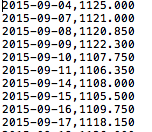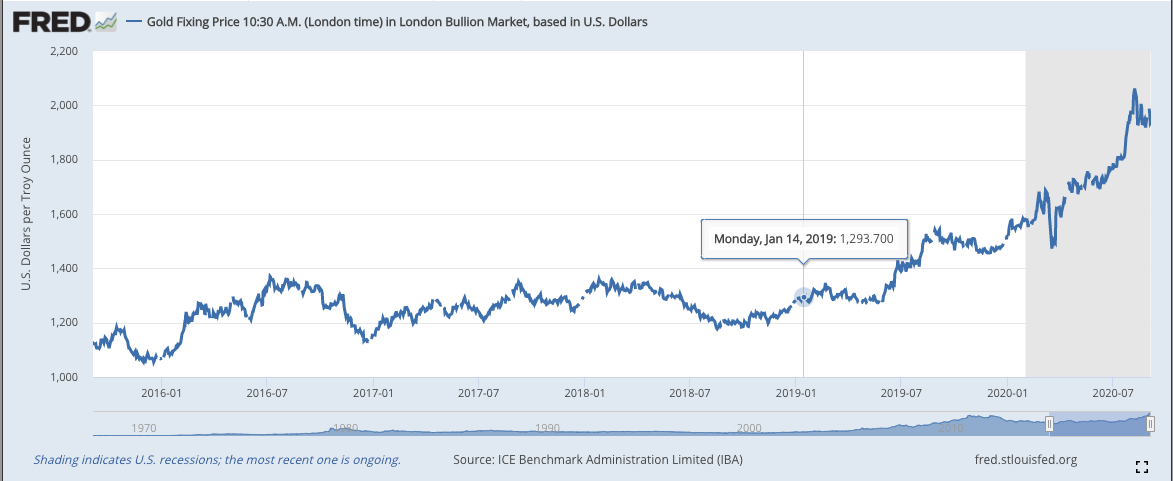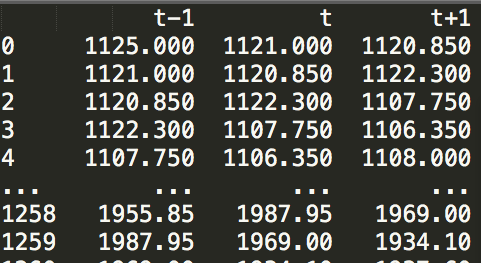# 背景概述# 数据滑动窗口原理1125，1121，120.85

1121，1120.85，1122.3

1120.85，1122.3，1107.75

........

# 代码示例from pandas import DataFrame

df = DataFrame()

getTex=open('goldPrice.csv')

rawData=[]

index=line.strip().split(',')

if index=='.':

continue   #filter dirty data

rawData.append(index)

df['t-1'] = rawData

df['t'] = df['t-1'].shift(-1)

df['t+1'] = df['t-1'].shift(-2)

print(df)Python Free

QQ：417803890